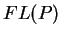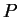Next: What is a dual Up: Convex Polyhedron Previous: What are the faces   Contents

## What is the face lattice of a convex polytope

The face posetof a convex polyhedron is the set of all faces ofordered by set inclusion. Two polytopes are called isomorphic if their face posets are isomorphic. The face poset of a convex polytope is a lattice.

The face poset of a convex polyhedron is sometimes referred to as the combinatorial structure of the polyhedron. Thus the expression ``two polyhedra are combinatorially equal'' means they are isomorphic.

Komei Fukuda 2004-08-26